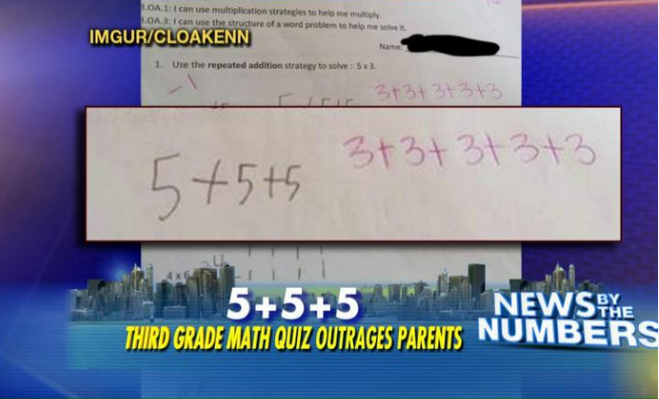# Teaching Arithmetic and Common Core

Teaching arithmetic under Common Core Standards—teaching arithmetic at all—requires the teacher first to understand arithmetic. Teaching any subject requires the teacher first to understand that subject, come to that, but in this article, I’m interested in arithmetic.

Below is an image from a Fox News Insider piece about a third-grader’s math problem: solve the problem “5 x 3 = ?.” The student wrote his answer in pencil; the teacher marked the answer wrong and wrote his version of the correct answer in red.Of course, anyone with an actual third grade education knows that both versions are correct. What’s the problem here?

The Common Core State Standards for Mathematics has this suggestion for teaching a third-grade multiplication problem:

1.  Interpret products of whole numbers, e.g., interpret 5 × 7 as the total number of objects in 5 groups of 7 objects each.

Thus, 5 x 3 might be interpreted as five groups of three objects each, as our student’s teacher has written. Except that by marking the student’s representation of three groups of five objects each as wrong, the teacher has shown he’s interpreting Common Core’s suggestion as a requirement, as the only right way to solve the problem.

The fault here is not in Common Core Standards; those are just setting a performance standard and suggesting a mechanism for achieving it.  No, the failure here is in the shocking arithmetic illiteracy—innumeracy—of this person presuming to “teach” third-graders their numbers.